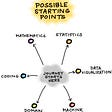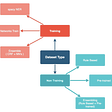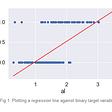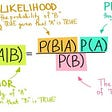# Batch Gradient Ascent and Logistic Regression

For this blog, I will explain logistic regression and how the beta values can be calculated through gradient ascent of the maximum log-likelihood.

Logistic regression is widely used for binary classifications where the dependant variables are limited to be either 0 or 1. Therefore, our predicted values should also range between 0 and 1 with a 50% threshold. This is to say, if the model returns a value greater than or equal to 50% then it will be classified as 1, and if less than 50, classified as 0.

Logistic regression is very similar to linear regression as they are both additive models. However, the values output values should range from [0, 1]. A common way to return this value is to utilize a sigmoid function.

The probability of y to be 1 given x variables parameterized by the theta(feature) values will then equal h_{\theta}(x). And because y can only be 0 or 1 the probability of y to be 0 given x variables parameterized by theta will equal 1 — h_{\theta}(x).

The likelihood of the theta(all parameters, variables) is the product of the probability of the data.

The objective is to now choose theta values that will maximize the log-likelihood. Very similarly to gradient descent, I will update each beta values simultaneously by taking the partial derivative. However, since I am now trying to climb to the top I will add the partial derivative of the log-likelihood instead of subtracting.

I hope this was helpful in understanding gradient ascent and its application to logistic regression.

--

--

--

## More from Albert Um

Hello! My name is Albert Um.

Love podcasts or audiobooks? Learn on the go with our new app.

## Getting Started with Data Science from scratch.## Struggling with micro-optimizations on large scale data processing## PB & J, Cookies n’ Cream — Pandas and SQLite3## Big Data Analytics Solutions and Services for the Enterprises Resources## Strategies for Learning Data Science## The Different Galaxy Project Communities Worldwide## Technology Extraction on People’s Professional Summary## Udacity Data Visualization Nanodegree Capstone Project## Albert Um

Hello! My name is Albert Um.

## Logistic Regression — In Depth Intuition with an example## Explaining Linear Regression## Gini vs Entropy : how do they find the optimum split?## Naive Bayes in Machine Learning